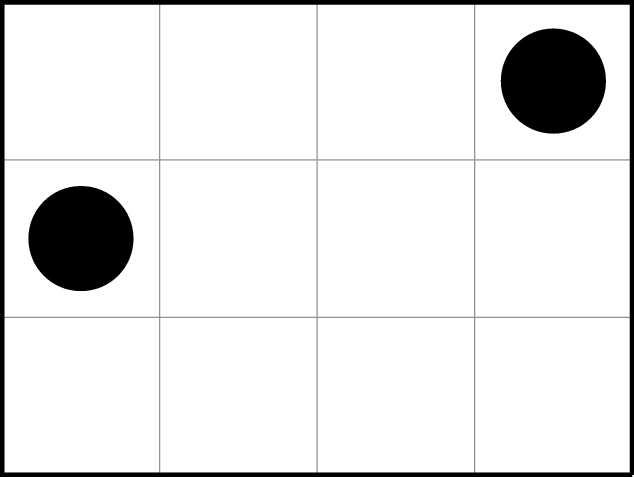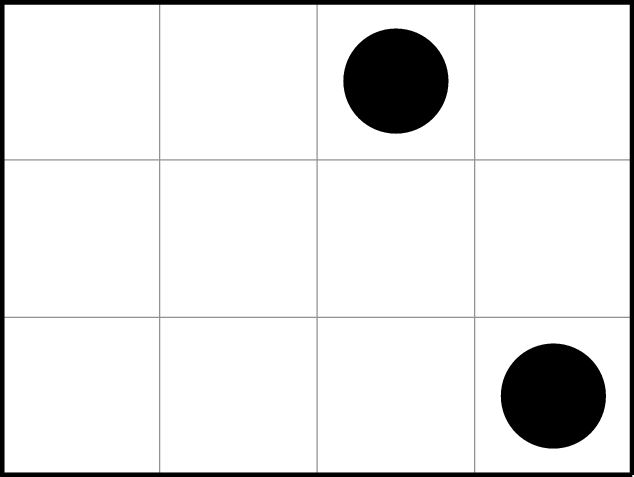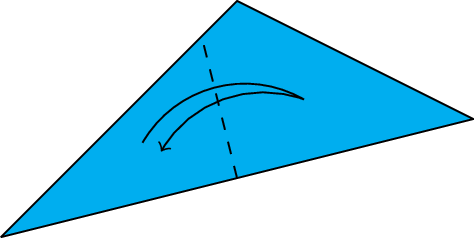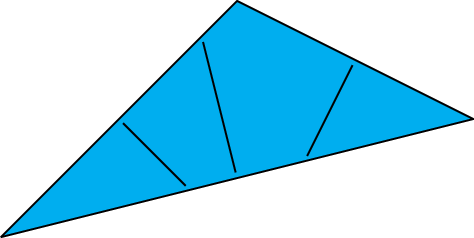## 2016 Qualifying Quiz

You may also be interested in the printable (PDF) format or the LaTeX source code.

1. It is the future, and you are working as an alchemist in the depths of the Earth's core. You work in the business of turning silver into gold and back. Each silver piece is worth 1 dollar; each gold piece is worth Φ = (1 +  5 ) / 2 dollars. You start with a single piece of silver. On your first day of the job, you turn the silver piece into a gold piece. On each successive day, you turn each silver piece from the previous day into a gold piece, and each gold piece from the previous day into two pieces, one silver and one gold. So at the end of day 1, you have 1 gold piece; at the end of day 2, you have 1 gold piece and 1 silver piece; at the end of day 3, you have 2 gold pieces and 1 silver piece.

Prove that at the end of day n, your treasure is worth Φn dollars for all positive integers n.
2. Francisco and Savannah are playing a game with two tokens, which are placed on the squares of a rectangular grid of arbitrary size, as shown in either part of Figure 1. The two tokens must be in different rows and columns. The players take turns moving a token of their choice to a different square, satisfying the following constraints:
• Tokens can never be moved upward or to the right.
• The row ordering must be preserved: if one token is above the other, it must stay above the other.
• The column ordering must be preserved: if one token is to the right of the other, it must stay to the right of the other.
Francisco goes first. Whichever player has no legal moves (with either token) loses.
1. Suppose one token begins above and to the right of the other, as in Figure 1(a). (The constraints require that the two tokens stay in that order.) For which starting positions does Francisco win and for which does Savannah win? What is the winning strategy in each case?
2. Do the same analysis assuming one token begins below and to the right of the other, as in Figure 1(b). (The constraints require that the two tokens stay in that order.)Figure 1(a): One token is above and to the right of the other. Figure 1(b): One token is below and to the right of the other.
3. Given an arbitrary triangle cut out from paper, we can fold one of its sides in half, so that one corner overlaps with another. This makes a crease through the midpoint of that side, as in Figure 2(a).Figure 2(a): Folding a triangle in half. Figure 2(b): A triangle with all three creases.
Unfolding and repeating with the other two sides, we get two more creases, as in Figure 2(b).
1. If two of the three creases have the same length, must the triangle be isosceles?
2. If all three creases have the same length, must the triangle be equilateral?
4. Drake is thinking of a positive integer x. He tells Misha the number of digits x has in base 2. He tells Ivy the number of digits x has in base 3. For example, if Drake thinks of x = 11 = 10112 = 1023, he'll tell Misha "x has 4 digits in base 2" and he'll tell Ivy "x has 3 digits in base 3".
1. Drake alternates asking Misha and Ivy if they know x. They have the following conversation:
Misha: No, I don't know x.
Ivy: No, I don't know x.
Misha: Yes, now I know x.
Ivy: Yes, now I know x.
What was x?
2. Suppose Drake instead chooses some other functions f and g, tells Misha f (x), and tells Ivy g (x). Drake then alternates asking them if they know x until they both say "Yes". The functions f and g are common knowledge: you, Misha, and Ivy all know what they are. But of course, you don't know the particular numbers f (x) and g (x) that Drake tells Misha and Ivy.

Can Drake choose functions f and g such that you can always deduce x just by listening to Misha and Ivy's conversation?
5. We call some positive integers oddly nice according to the following rules:
• 1 is oddly nice.
• An integer n > 1 is oddly nice if and only if an odd number of its proper divisors are oddly nice.
Which numbers are oddly nice? If s(n) is the number of oddly nice proper divisors of an integer n, what are all the possible values of s(n)? Prove your answer.

You might find the following background reading useful: www.cut-the-knot.org/blue/NumberOfFactors.shtml.
6. Waley starts with a list of all the positive integers in order. He can perform the following operations on it:
• A 2-flip, which reverses pairs of elements, turning 1, 2, 3, 4, 5, 6, … into 2, 1, 4, 3, 6, 5, … .
• A 3-flip, which reverses triples of elements, turning 1, 2, 3, 4, 5, 6, … into 3, 2, 1, 6, 5, 4, … .
• More generally, an n-flip, for any integer n > 1: the list is split into groups of n consecutive terms, and then each group is reversed.
Waley can perform any number of these operations, in any order. For instance, he can perform a 2-flip and then a 3-flip, which will first turn 1, 2, 3, 4, 5, 6, 7, 8, … into 2, 1, 4, 3, 6, 5, 8, 7, … and then into 4, 1, 2, 5, 6, 3, 10, 7, 8, … .

If you give Waley a finite sequence of distinct positive integers, when can he put that sequence at the beginning of his list (in order)? You should find a strategy for Waley to follow whenever this can be done, and prove that all other sequences are not attainable.

Problem #2 is due to Palmer Mebane, MC '07-'08; problem #3 is due to Waley Zhang, MC '14-'15; problems #4 and #6 are due to Drake Thomas, MC '14-'15; problems #1 and #5 were written by the Mathcamp staff.